Setup for 5 Player a Game of Food Chain Magnate

Originally generated on 1/11/2019 12:26:27 AM

Player Setup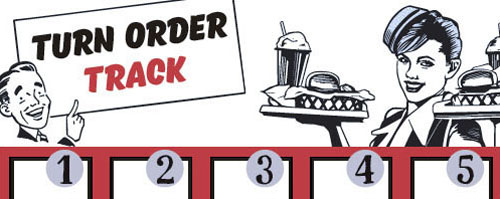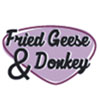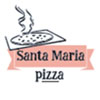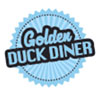Other Setup

Bank starts with \$250.00
Number of 1x Employee cards used = 3

Map (Random)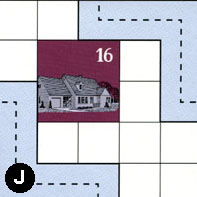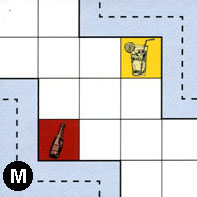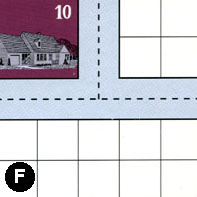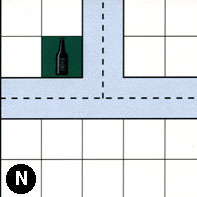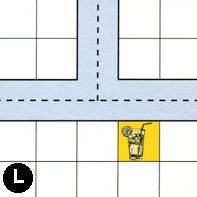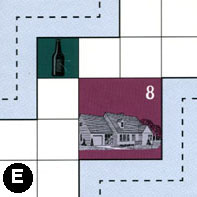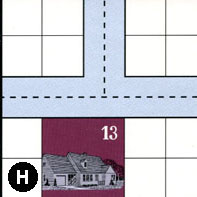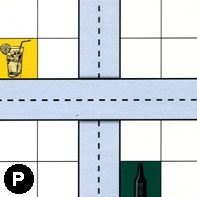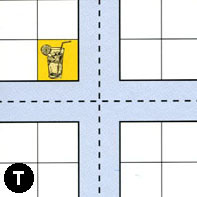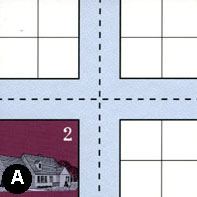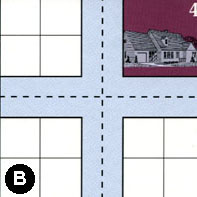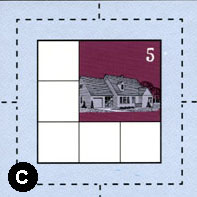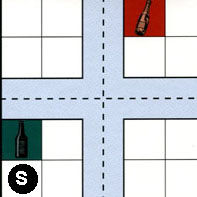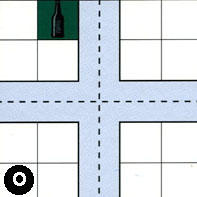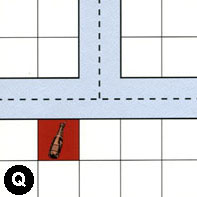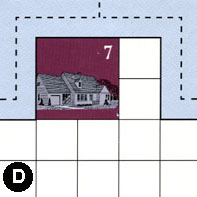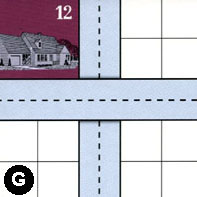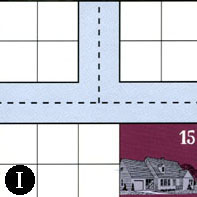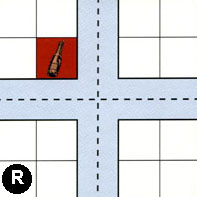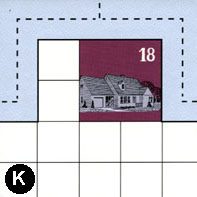Map Stats

Map Option = Random
Total Number of Tiles = 20
Total Number of Starting Houses = 11
Total Number of Beer Spots = 5
Total Number of Soda Spots = 4
Total Number of Lemonade Spots = 4
Total Number of Drink Spots = 13
Number of independent path systems = 3
Number of independent neighborhoods = 18

Path System 1
Size = Tiny
Number of Tiles = 1
Number of Paths = 1
Contains Loop(s) = No
Number of Starting Houses = 1
Starting Houses Pct of Map (%) = 9
Number of Beer Spots = 0
Number of Soda Spots = 0
Number of Lemonade Spots = 0
Number of Drink Spots = 0
Drink Spots Pct of Map (%) = 0
Tile Ids = J
Paths = JWS What do these mean?
Starting House Ids = 16
Path System 2
Size = Small
Number of Tiles = 2
Number of Paths = 4
Contains Loop(s) = No
Number of Starting Houses = 2
Starting Houses Pct of Map (%) = 18
Number of Beer Spots = 0
Number of Soda Spots = 0
Number of Lemonade Spots = 1
Number of Drink Spots = 1
Drink Spots Pct of Map (%) = 8
Tile Ids = F, M
Paths = MEN, FWN, FNE, FWE What do these mean?
Starting House Ids = 0, 10
Path System 3
Size = Large
Number of Tiles = 19
Number of Paths = 71
Contains Loop(s) = No
Number of Starting Houses = 11
Starting Houses Pct of Map (%) = 100
Number of Beer Spots = 5
Number of Soda Spots = 4
Number of Lemonade Spots = 3
Number of Drink Spots = 12
Drink Spots Pct of Map (%) = 92
Tile Ids = A, B, C, D, E, G, H, I, J, K, L, M, N, O, P, Q, R, S, T
Paths = NWN, LWE, LWN, AWE, AWN, TWE, PWE, HWN, MWS, JEN, EWN, HWE, CWS, SWS, PNS, SWN, OWN, RWE, RWN, RNE, OWE, TWS, TWN, NWE, NNE, LNE, ANE, TNE, TNS, ONE, SNE, IWE, IWN, GNS, INE, SWE, SES, CWN, GWE, CNE, BWN, EES, BWE, DWE, DWN, DNE, BNE, CWE, SNS, ONS, QWE, QWN, KWN, RWS, RNS, RES, OWS, QNE, KNE, KWE, OES, TES, ANS, AWS, AES, CES, HNE, CNS, BNS, BWS, BES What do these mean?
Starting House Ids = 0, 2, 4, 5, 7, 8, 12, 13, 15, 16, 18

Neighborhood 1
Total Size = Huge
Number of Total Spaces = 99
Number of Empty Spaces (for new houses & gardens) = 82
Number of Beer Spots = 1
Number of Soda Spots = 1
Number of Lemonade Spots = 3
Number of Drink Spots = 5
Number of Starting Houses = 3
Starting Houses Pct of Map (%) = 27
Tile Ids = B, E, F, H, J, M, N, P, T
Starting House Ids = 8, 13, 16
Neighborhood 2
Total Size = Small
Number of Total Spaces = 4
Number of Empty Spaces (for new houses & gardens) = 0
Number of Beer Spots = 0
Number of Soda Spots = 0
Number of Lemonade Spots = 0
Number of Drink Spots = 0
Number of Starting Houses = 1
Starting Houses Pct of Map (%) = 9
Tile Ids = F
Starting House Ids = 10
Neighborhood 3
Total Size = Medium
Number of Total Spaces = 14
Number of Empty Spaces (for new houses & gardens) = 12
Number of Beer Spots = 1
Number of Soda Spots = 0
Number of Lemonade Spots = 1
Number of Drink Spots = 2
Number of Starting Houses = 0
Starting Houses Pct of Map (%) = 0
Tile Ids = L, N
Neighborhood 4
Total Size = Medium
Number of Total Spaces = 16
Number of Empty Spaces (for new houses & gardens) = 16
Number of Beer Spots = 0
Number of Soda Spots = 0
Number of Lemonade Spots = 0
Number of Drink Spots = 0
Number of Starting Houses = 0
Starting Houses Pct of Map (%) = 0
Tile Ids = A, L, N, T
Neighborhood 5
Total Size = Medium
Number of Total Spaces = 8
Number of Empty Spaces (for new houses & gardens) = 8
Number of Beer Spots = 0
Number of Soda Spots = 0
Number of Lemonade Spots = 0
Number of Drink Spots = 0
Number of Starting Houses = 0
Starting Houses Pct of Map (%) = 0
Tile Ids = A, L
Neighborhood 6
Total Size = Medium
Number of Total Spaces = 12
Number of Empty Spaces (for new houses & gardens) = 11
Number of Beer Spots = 1
Number of Soda Spots = 0
Number of Lemonade Spots = 0
Number of Drink Spots = 1
Number of Starting Houses = 0
Starting Houses Pct of Map (%) = 0
Tile Ids = H, P, S
Neighborhood 7
Total Size = Medium
Number of Total Spaces = 16
Number of Empty Spaces (for new houses & gardens) = 15
Number of Beer Spots = 1
Number of Soda Spots = 0
Number of Lemonade Spots = 0
Number of Drink Spots = 1
Number of Starting Houses = 0
Starting Houses Pct of Map (%) = 0
Tile Ids = O, P, S, T
Neighborhood 8
Total Size = Large
Number of Total Spaces = 26
Number of Empty Spaces (for new houses & gardens) = 21
Number of Beer Spots = 0
Number of Soda Spots = 1
Number of Lemonade Spots = 0
Number of Drink Spots = 1
Number of Starting Houses = 1
Starting Houses Pct of Map (%) = 9
Tile Ids = A, O, Q, T
Starting House Ids = 2
Neighborhood 9
Total Size = Small
Number of Total Spaces = 4
Number of Empty Spaces (for new houses & gardens) = 4
Number of Beer Spots = 0
Number of Soda Spots = 0
Number of Lemonade Spots = 0
Number of Drink Spots = 0
Number of Starting Houses = 0
Starting Houses Pct of Map (%) = 0
Tile Ids = B
Neighborhood 10
Total Size = Medium
Number of Total Spaces = 9
Number of Empty Spaces (for new houses & gardens) = 5
Number of Beer Spots = 0
Number of Soda Spots = 0
Number of Lemonade Spots = 0
Number of Drink Spots = 0
Number of Starting Houses = 1
Starting Houses Pct of Map (%) = 9
Tile Ids = C
Starting House Ids = 5
Neighborhood 11
Total Size = Small
Number of Total Spaces = 4
Number of Empty Spaces (for new houses & gardens) = 4
Number of Beer Spots = 0
Number of Soda Spots = 0
Number of Lemonade Spots = 0
Number of Drink Spots = 0
Number of Starting Houses = 0
Starting Houses Pct of Map (%) = 0
Tile Ids = B
Neighborhood 12
Total Size = Large
Number of Total Spaces = 28
Number of Empty Spaces (for new houses & gardens) = 20
Number of Beer Spots = 0
Number of Soda Spots = 0
Number of Lemonade Spots = 0
Number of Drink Spots = 0
Number of Starting Houses = 2
Starting Houses Pct of Map (%) = 18
Tile Ids = B, D, G
Starting House Ids = 4, 7
Neighborhood 13
Total Size = Medium
Number of Total Spaces = 12
Number of Empty Spaces (for new houses & gardens) = 8
Number of Beer Spots = 0
Number of Soda Spots = 0
Number of Lemonade Spots = 0
Number of Drink Spots = 0
Number of Starting Houses = 1
Starting Houses Pct of Map (%) = 9
Tile Ids = G, I, S
Starting House Ids = 12
Neighborhood 14
Total Size = Large
Number of Total Spaces = 26
Number of Empty Spaces (for new houses & gardens) = 19
Number of Beer Spots = 1
Number of Soda Spots = 2
Number of Lemonade Spots = 0
Number of Drink Spots = 3
Number of Starting Houses = 1
Starting Houses Pct of Map (%) = 9
Tile Ids = I, O, R, S
Starting House Ids = 15
Neighborhood 15
Total Size = Medium
Number of Total Spaces = 12
Number of Empty Spaces (for new houses & gardens) = 12
Number of Beer Spots = 0
Number of Soda Spots = 0
Number of Lemonade Spots = 0
Number of Drink Spots = 0
Number of Starting Houses = 0
Starting Houses Pct of Map (%) = 0
Tile Ids = O, Q, R
Neighborhood 16
Total Size = Small
Number of Total Spaces = 4
Number of Empty Spaces (for new houses & gardens) = 4
Number of Beer Spots = 0
Number of Soda Spots = 0
Number of Lemonade Spots = 0
Number of Drink Spots = 0
Number of Starting Houses = 0
Starting Houses Pct of Map (%) = 0
Tile Ids = Q
Neighborhood 17
Total Size = Medium
Number of Total Spaces = 20
Number of Empty Spaces (for new houses & gardens) = 16
Number of Beer Spots = 0
Number of Soda Spots = 0
Number of Lemonade Spots = 0
Number of Drink Spots = 0
Number of Starting Houses = 1
Starting Houses Pct of Map (%) = 9
Tile Ids = K, R
Starting House Ids = 18
Neighborhood 18
Total Size = Medium
Number of Total Spaces = 8
Number of Empty Spaces (for new houses & gardens) = 8
Number of Beer Spots = 0
Number of Soda Spots = 0
Number of Lemonade Spots = 0
Number of Drink Spots = 0
Number of Starting Houses = 0
Starting Houses Pct of Map (%) = 0
Tile Ids = G, I

v2.1.5